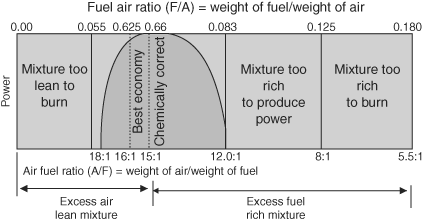# air-fuel mixture ratio

﻿
air-fuel mixture ratio
The ratio by weight of air and fuel in the mixture being sent into the cylinders of a reciprocating engine. Ideally, the ratio should be 15:1. Besides the ideal (stoichiometric) ratio, the air-fuel mixture may be rich or lean depending on the quantity of fuel in the mixture. With more fuel, the mixture is rich, and with less fuel, it is a weak or lean mixture.Aviation dictionary. 2014.

### Look at other dictionaries:

• lean air-fuel mixture — A mixture of air and fuel in which there is more air and less fuel. See air fuel ratio …   Dictionary of automotive terms

• rich air-fuel mixture — A mixture of air and fuel in which there is less air and more fuel. See air fuel ratio …   Dictionary of automotive terms

• Air-fuel ratio — (AFR) is the mass ratio of air to fuel present during combustion. When all the fuel is combined with all the free oxygen, typically within a vehicle s combustion chamber,the mixture is chemically balanced and this AFR is called the stoichiometric …   Wikipedia

• Air-fuel ratio meter — An air fuel ratio meter monitors the air fuel ratio of an internal combustion engine. Also called air fuel ratio gauge, air fuel meter, or air fuel gauge. It reads the voltage output of an oxygen sensor, sometimes also called lambda sensor,… …   Wikipedia

• air-fuel ratio — (A/F ratio) The mass of air supplied to the engine divided by the mass of fuel supplied in the same period of time. The stoichiometric, or chemically correct, air fuel ratio is the exact ratio necessary to burn all the carbon and hydrogen in the… …   Dictionary of automotive terms

• Fuel injection — Fuel rail connected to the injectors that are mounted just above the intake manifold on a four cylinder engine. Fuel injection …   Wikipedia

• Fuel cell — For other uses, see Fuel cell (disambiguation). Demonstration model of a direct methanol fuel cell. The actual fuel cell stack is the layered cube shape in the center of the image A fuel cell is a device that converts the chemical energy from a… …   Wikipedia

• ratio — A fixed relationship between things in number, quantity or degree. For example, if the fuel mixture contains one part of gas for fifteen parts of air, the ratio would be 15 to 1. See a/f ratio a/f ratio air fuel ratio air fuel ratio air ratio… …   Dictionary of automotive terms

• fuel — A combustible material used to produce energy. One of the essential factors in a combustion engine (Fuel, Air, Proper proportion of mixture, compression, timing, spark). Also see air fuel ratio aviation fuel deceleration fuel cut off diesel fuel… …   Dictionary of automotive terms

• Fuel mass fraction — In combustion physics, fuel mass fraction is the ratio of fuel mass flow to the total mass flow of a fuel mixture. If an air flow is fuel free, the fuel mass fraction is zero; in pure fuel without trapped gases, the ratio is unity. [cite book |… …   Wikipedia